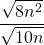# 9.4 Multiplication and Division of Radicals

Multiplying radicals is very simple if the index on all the radicals match. The product rule of radicals, which is already been used, can be generalized as follows: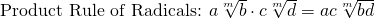This means that, if the index on the radicals match, then simply multiply the factors outside the radical and also multiply the factors inside the radicals. An example showing this is as follows.

Example 9.4.1

Multiply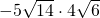.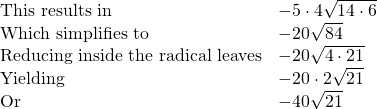This same process works with any higher root radicals having matching indices.

Example 9.4.2

Multiply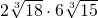.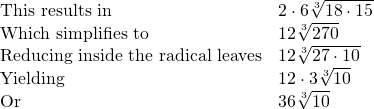Example 9.4.3

Multiply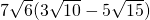.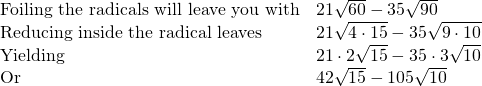Example 9.4.4

Multiply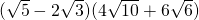.

Multiplying the factors inside and outside the radicals yields: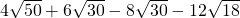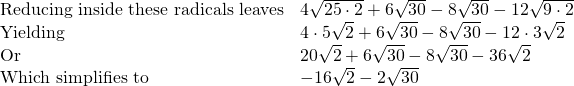Division with radicals is very similar to multiplication. If you think about division as reducing fractions, you can reduce the coefficients outside the radicals and reduce the values inside the radicals to get our final solution. There is one catch to dividing with radicals: it is considered bad practice to have a radical in the denominator of a final answer, so if there is a radical in the denominator, it should be rationalized by cancelling or multiplying the radicals.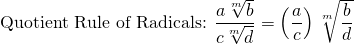The quotient rule means that factors outside the radical are divided by each other and the factors inside the radical are also divided by each other. To see this illustrated, consider the following:

Example 9.4.5

Reduce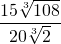.

Using the quotient rule of radicals, this problem is separated into factors inside and outside the radicals. This results in the following: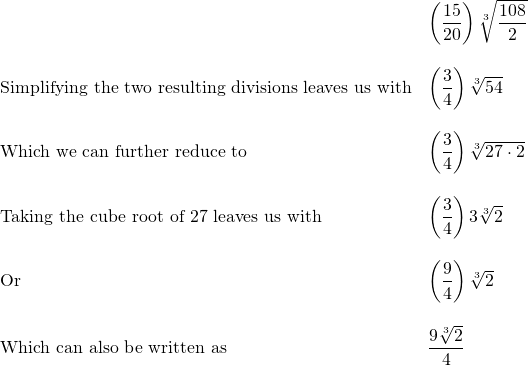Removing radicals from the denominator that cannot be divided out by using the numerator is often simply done by multiplying the numerator and denominator by a common radical. This is easily done and is shown by the following examples.

Example 9.4.6

Rationalize the denominator of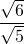.

For this pair of radicals, the denominator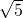cannot be cancelled by the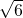, so the solution requires thatbe rationalized through multiplication. This is done as follows: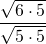This now simplifies to: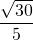This process is similar for radicals in which the index is greater than 2.

Example 9.4.7

Rationalize the denominator of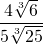.

To rationalize the denominator, we need to get a cube root of 125, which will leave us with a denominator of 5 × 5. This requires that both the numerator and the denominator to be multiplied by the cube root of 5. This looks like: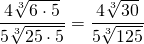This simplifies to: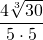Or: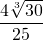The last example to be considered involves rationalizing denominators that have variables. Remeber to always reduce any fractions (inside and outside of the radical) before rationalizing.

Example 9.4.8

Rationalize the denominator of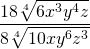.

The first thing to do is cancel all common factors both inside and outside the radicals. This leaves: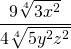The next step is to multiply both the numerator and denominator to rationalize the denominator: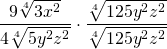Multiplying these yields: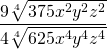Taking the fourth root of the denominator leaves: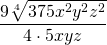Or: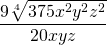# Questions

Simplify.

1.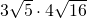2.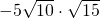3.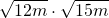4.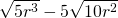5.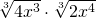6.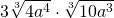7.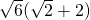8.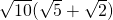9.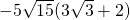10.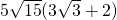11.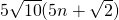12.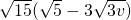13.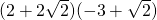14.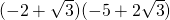15.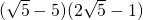16.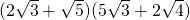17.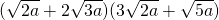18.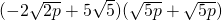19.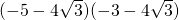20.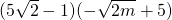21.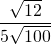22.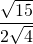23.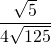24.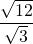25.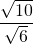26.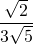27.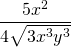28.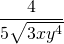29.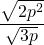30.